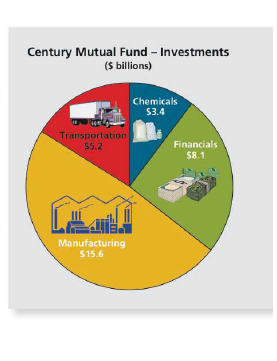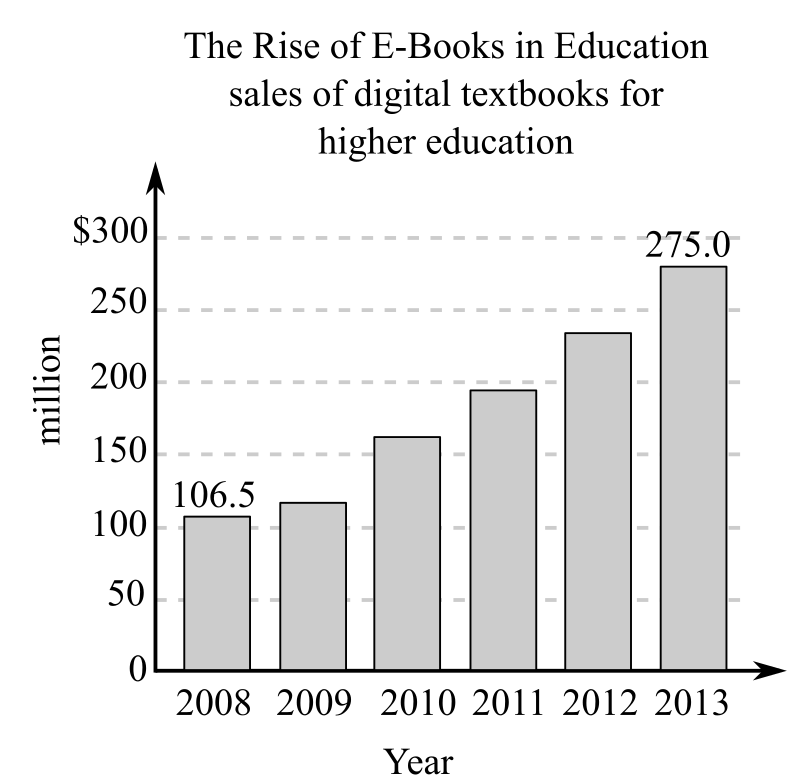Chapter 6, Problem 48AT### Contemporary Mathematics for Busin...

8th Edition
Robert Brechner + 1 other
ISBN: 9781305585447

#### Solutions

Chapter
Section### Contemporary Mathematics for Busin...

8th Edition
Robert Brechner + 1 other
ISBN: 9781305585447
Textbook Problem

# Use the chart “The Rise of E-Books in Education” for Exercises 47-49.What were the sales of education e-books in 2009 if they were 10.3% higher than 2008? Round to the nearest tenth of a million.To determine

To calculate: The amount of sales of education e-books in 2009 by using chart and also round it to nearest tenth of a million such that the sales were 10.3% higher sales than 2008. The chart is as follows:Explanation

Given Information:

The sales were 10.3% higher than 2008 in 2009 and the provided chart is as follows:

Formula used:

Steps for computing percentage problems;

Step1: Recognize the two known values and the unknown value.

Step2: Pick the formula that solves the unknown value.

Step3: Solve the equation by putting the known values for the letters in the formula.

The formula to compute portion is;

P=R×B

Calculation:

Consider that the original value or the base is \$106.5 million and rate of increase is 10.3%.

As the rate of change is an increase, hence add that change to 100% so as to compute the rate,

R=(100%+10.3%)=110.3%

Hence rate is R=110

### Still sussing out bartleby?

Check out a sample textbook solution.

See a sample solution

#### The Solution to Your Study Problems

Bartleby provides explanations to thousands of textbook problems written by our experts, many with advanced degrees!

Get Started